# ALPHY5: MECHANICS

##### This unit explains mechanics in Physics

Physical Quantities And Their Units

The International System of Units (abbreviated as SI Units from its French name, Système International d’unités) is an internationally agreed metric system of units of measurement that has been in existence since 1960.  The system itself is based on the concept of seven fundamental base units of quantity, from which all other units of quantity can be derived. Following the end of the Second World War, it became increasingly apparent that a worldwide system of measurement was needed to replace the numerous and diverse systems of measurements in use at that time. In 1954, the 10th General Conference on Weights and Measures, acting on the findings of an earlier study, proposed a system based on six base quantities.The quantities recommended the metrekilogramsecondamperekelvin and candela.

S1 Base Units And Quantities

The value of a physical quantity is usually expressed as the product of a number and a unit. The unit represents a specific example or prototype of the quantity concerned, which is used as a point of reference. The number represents the ratio of the value of the quantity to the unit. In the case of the kilogram, the prototype is a platinum-iridium cylinder held under tightly controlled conditions in a vault at the BIPM, although there are a number of identical copies kept under identical conditions located throughout the world. A quantity of two kilograms (2 kg) would have exactly twice the mass of the prototype or one of its copies. There are seven base quantities used in the International System of Units. The seven base quantities and their corresponding units are:

• length (metre)
• mass (kilogram)
• time (second)
• electric current (ampere)
• thermodynamic temperature (kelvin)
• amount of substance (mole)
• luminous intensity (candela)

These base quantities are assumed to be independent of one another. In other words, no base quantity needs to be defined in terms of any other base quantity (or quantities). Note however that although the base quantities themselves are considered to be independent, their respective base units are in some cases dependent on one another. The metre, for example, is defined as the length of the path travelled by light in a vacuum in a time interval of 1/299 792 458 of a second. The table below summarizes the base quantities and their units. You may have noticed that an anomaly arises with respect to the kilogram (the unit of mass). The kilogram is the only SI base unit whose name and symbol include a prefix. You should be aware that multiples and submultiples of this unit are formed by attaching the appropriate prefix name to the unit name gram, and the appropriate prefix symbol to the unit symbol g. For example, one millionth of a kilogram is one milligram (1 mg), and not one microkilogram (1 μkg).

SI Base Units
Qty Sym. Unit Unit
Sym.
Unit Definition
length l metre m The length of the path travelled by light in 1/299 792 458of a second
mass m kilogram kg The mass of the International Prototype Kilogram
time t second s The duration of 9 192 631 770 periods of the radiation corresponding to the transition between the two hyperfine levels of the ground state of the caesium 133 atom, at rest, at a temperature of 0 K
electric
current
I ampere A The constant current which, if maintained in two straight parallel conductors of infinite length, of negligible circular cross-section, and placed one metre apart in a vacuum, would produce between these conductors a force equal to 2 x 10-7 newton per metre of length
thermodynamic
temperature
T kelvin K The fraction 1/273.16 of the thermodynamic temperature of the triple point of water
amount
of
substance
n mole mol The amount of substance of a system which contains as many elementary entities as there are atoms in 0.012kilogram of carbon 12 (elementary entities, which must be specified, may be atoms, molecules, ions, electrons, other particles or specified groups of such particles)
luminous
intensity
Iv candela cd The luminous intensity, in a given direction, of a source that emits monochromatic radiation of frequency 540 × 1012hertz and that has a radiant intensity in that direction of 1/683 watts per steridian

Coherent Derived Units Expressed In Terms of S1 Base Units

The derived units of quantity identified by the International System of Units are all defined as products of powers of base units. A derived quantity can therefore be expressed in terms of one or more base quantity in the form of an algebraic expression. Derived units that are products of powers of base units that include no numerical factor other than one are said to be coherent derived units. This means that they are derived purely using products or quotients of integer powers of base quantities, and that no numerical factor other than one is involved. The table below gives some examples of coherent derived units.

SI Derived Units expressed in terms of Base Units
Qty Sym. Unit Unit
Sym.
area A square metre m2
volume V cubic metre m3
speed, velocity v metre per second m/s
acceleration a metre per second squared m/s2
wavenumber σ reciprocal metre m-1
density, mass density ρ kilogram per cubic metre kg/m3
surface density ρA kilogram per square metre kg/m2
specific volume v cubic metre per kilogram m3/kg
current density j ampere per square metre A/m2
magnetic field strength H ampere per metre A/m
amount concentration, concentration c mole per cubic metre mol/m3
mass concentration ρ kilogram per cubic metre kg/m3
luminance Lv candela per square metre cd/m2
refractive index n one 1
relative permeability μr one 1

Coherent Derived Units With Special Units And Symbols

There are a number of derived SI units that have special names and symbols. Often, the name chosen acknowledges the contribution of a particular scientist. The unit of force (the newton) is named after Sir Isaac Newton, one of the greatest contributors in the field of classical mechanics. The unit of pressure (the pascal) is named after Blaise Pascal for his work in the fields of hydrodynamics and hydrostatics. Each unit named in the table below has its own symbol, but can be defined in terms of other derived units or in terms of the SI base units, as shown in the last two columns.

Note that the units for the plane angle and the solid angle (the radian and steradian respectively) are both derived as the quotient of two identical SI base units. They are thus said to have the unit one (1). They are described as dimensionless units or units of dimension one (the concept of dimension will be described shortly). Note that a temperature difference of one degree Celsius has exactly the same value as a temperature difference of one kelvin. The Celsius temperature scale tends to be used for day-to-day non-scientific purposes such as reporting the weather, or for specifying the temperature at which foodstuffs and medicines should be stored. In this kind of context it is somewhat more meaningful to a member of the public than the Kelvin temperature scale.

SI Derived Units with special names
Qty Unit Unit Sym. Other
Units
Base
Units
plane angle radian rad 1 m/m
solid angle steradian sr 1 m2/m2
frequency hertz Hz s-1
force newton N m kg s-2
pressure,
stress
pascal Pa N/m2 m-1 kg s-2
energy,
work,
amount of heat
joule J N m m2 kg s-2
power,
radiant flux
watt W J/s m2 kg s-3
electric charge,
amount of electricity
coulomb C s A
electric potential difference,
electromotive force
volt V W/A m2 kg s-3 A-1
capacitance farad F C/V m-2 kg-1 s4 A2
electric resistance ohm Ω V/A m2 kg s-3 A-2
electric conductance siemens S A/V m-2 kg-1 s3 A2
magnetic flux weber Wb V s m2 kg s-2 A-1
magnetic flux density tesla T Wb/m2 kg s-2 A-1
inductance henry H Wb/A m2 kg s-2 A-2
Celsius temperature degree Celsius °C K
luminous flux lumen lm cd sr cd
illuminance lux lx lm/m2 m-2 cd
activity referred to a radio nuclide becquerel Bq s-1
absorbed dose,
specific energy (imparted),
kerma
gray Gy J/kg m2 s-2
dose equivalent,
ambient dose equivalent,
directional dose equivalent,
personal dose equivalent
sievert Sv J/kg m2 s-2
catalytic activity katal kat s-1 mol

## Coherent Derived Units With Hybrid Names and Symbols

The coherent SI derived units shown in the table below are based on a combination of derived units with special names and the SI base units. The names and symbols for these units reflects the hybrid nature of these units. As with the units in the previous table, each unit has its own symbol but can be defined in terms of the SI base units, as shown in the final column. The value of being able to use both special and hybrid symbols in equations can be appreciated when we look at the length of some of the base unit expressions.

SI Derived Units with hybrid names
Qty Unit Unit
Symbol
Base
Units
dynamic viscosity pascal second Pa s m-1 kg s-1
moment of force newton metre N m m2 kg s-2
surface tension newton per metre N/m kg s-2
angular velocity radian per second rad/s m m-1 s-1 = s-1
angular acceleration radian per second squared rad/s2 m m-1 s-2 = s-2
heat flux density,
irradiance
watt per square metre W/m2 kg s-3
heat capacity,
entropy
joule per kelvin J/K m2 kg s-2 K-1
Specific heat capacity,
specific entropy
joule per kilogram kelvin J/(kg K) m2 s-2 K-1
specific energy joule per kilogram J/kg m2 s-2
thermal conductivity watt per metre kelvin W/(m K) m kg s-3 K-1
energy density joule per cubic metre J/m3 m-1 kg s-2
electric field strength volt per metre V/m m kg s-3 A-1
electric charge density coulomb per cubic metre C/m3 m-3 s A
surface charge density coulomb per square metre C/m2 m-2 s A
electric flux density,
electric displacement
coulomb per square metre C/m2 m-2 s A
permittivity farad per metre F/m m-3 kg-1 s4 A2
permeability henry per metre H/m m kg s-2 A-2
molar energy joule per mole J/mol m2 kg s-2 mol-1
molar entropy,
molar heat capacity
joule per mole kelvin J/(mol K) m2 kg s-2 K-1 mol-1
exposure (x- and γ-rays) coulomb per kilogram C/kg kg-1 s A
absorbed dose rate gray per second Gy/s m2 s-3
radiant intensity watt per steradian W/sr m4 m-2 kg s-3 = m2 kg s-3
radiance watt per square metre steradian W/(m2 sr) m2 m-2 kg s-3 = kg s-3
catalytic activity concentration katal per cubic metre kat/m3 m-3 s-1 mol

## Non-SI units accepted for use with the International System of Units

The units detailed in the remaining four tables are accepted for use with the International System of Units for a variety of reasons. Many are still in use, some are required for the interpretation of scientific texts of historical importance, and some are used in specialised areas such as medicine. The hectare, for example, is still commonly used to express land area. The use of the equivalent SI units is preferred for modern scientific texts. Wherever reference is made to non-SI units, they should be cross referenced with their equivalent SI units. For the units shown in the following tables, the equivalent definition in terms of SI units is also shown. The first of these tables lists units that are still in widespread daily use, and likely to be so for the foreseeable future.

Note that for most purposes, it is recommended that fractional values for plane angles expressed in degrees should be expressed using decimal fractions rather than minutes and seconds. Exceptions include navigation and surveying (due to the fact that one minute of latitude on the Earth’s surface corresponds to approximately one nautical mile), and astronomy. In the field of astronomy, very small angles are significant due to the enormous distances involved. It is therefore convenient for astronomers to use a unit of measurement that can represent very small differences in angle in a meaningful way. Very small angles can be represented in terms of arcsecondsmicroarcseconds and picoarcseconds.

Non-SI Units still in widespread use
Qty Unit Unit
Symbol
SI
Units
time minute min 1 min = 60 s
time hour h 1 h = 60 min = 3600 s
time day d 1 d = 24 h = 86 400 s
plane angle degree ° 1° = (π/180) rad
plane angle minute 1′ = (1/60)° = (π/10 800) rad
plane angle second 1″ = (1/60)′ = (π/648 000) rad
area hectare ha 1 ha = 1 hm2 = 104 m2
volume litre L or l 1L = 1 dm3 = 103 cm3 = 10-3 m3
mass tonne t 1 t = 103 kg

The table below lists a number of units related to fundamental constants (with the exception of the astronomical unit). These non-SI units are used to express quantities in certain specialised fields of scientific endeavor because it is simply more convenient to do so than using the equivalent SI unit, or because their use provides a more meaningful expression of quantities in a given context. The astronomical unit, for example, represents the approximate mean distance from the Earth to the Sun (149 597 870 700 metres). The value of these units must be determined experimentally due to the fact that, for one reason or another, they represent quantities that cannot be measured directly using conventional means. The abbreviations n.u. and a.u. stand for natural unit and atomic unit respectively.

The very nature of the quantities involved means that there will be a degree of uncertainty associated with each of them. This degree of uncertainty (known as the standard uncertainty) is expressed as a number in parentheses immediately following the value of the unit, and represents the amount by which the last two digits may vary. From the table below, for example, we can see that the electronvolt has a value (in Joules) of 1.602 176 53 × 10-19 plus or minus 0.000 000 14 × 10-19. This means its actual value may lie between 1.602 176 39 × 10-19 and 1.602 176 67 × 10-19Joules.

Non-SI Units whose values are obtained experimentally
Qty Unit Unit
Sym.
SI
Units
energy electronvolt eV 1 eV = 1.602 176 53 (14) × 10-19 J
mass dalton Da 1 Da = 1.660 538 86 (28) × 10-27 kg
mass unified atomic mass unit u 1 u = 1.660 538 86 (28) × 10-27 kg
length astronomical unit ua 1 ua = 1.495 978 706 91 (6) × 1011 m
speed n.u. of speed (speed of light in a vacuum) c0 299 792 458 m/s (exact)
action n.u. of action (reduced Planck constant) h 1.054 571 68 (18) × 10-34 J s
mass n.u. of mass (electron mass) me 9.109 3826 (16) × 10-31 kg
time n.u. of time h/(mec02) 1.288 088 6677 (86) × 10-21 s
charge a.u. of charge e 1.602 176 53 (14) × 10-19 C
mass a.u. of mass (electron mass) me 9.109 3826 (16) × 10-31 kg
action a.u. of action (reduced Planck constant) h 1.054 571 68 (18) × 10-34 J s
length a.u. of length, bohr (Bohr radius) a0 0.529 177 2108 (18) × 10-10 m
energy a.u. of energy, hartree (Hartree energy) Eh 4.359 744 17 (75) × 10-18 J
time a.u. of time h/Eh 2.418 884 326 505 (16) × 10-17 s

The next table represents units that have precisely defined SI equivalents, but that are used by particular organisations of groups due to commercial or legal reasons, or because (again) they are simply more convenient or meaningful in a particular context. For example, the nautical mile is used to express the distances travelled by ships and aircraft, while the knot (or nautical mile per hour) is used to express their speeds. A nautical mile is equal to 1852 metres, and represents approximately one minute of latitude. Note in particular the three dimensionless units (the neperbel and decibel) which represent logarithmic ratio quantities. The decibel (dB) is one tenth of a bel, and is probably the most frequently encountered of these units. In the field of telecommunications, the decibel is typically used to express signal strength relative to some specified reference level. For example, signal-to-noise ratio (SNR) is expressed using a decibel value of 10 log10 S/N. A signal-to-noise ratio of 10 would be expressed as 10 dB, a ratio of 100 as 20 dB, a ratio of 1000 as 30 dB and so on.

Other Non-SI Units
Qty Unit Unit
Symbol
SI
Units
pressure bar bar 1 bar = 0.1 MPa = 100 kPa = 105 Pa
pressure millimeter of mercury mmHg 1 mmHg ≈ 133.322 Pa
length ångström Å 1 Å = 0.1 nm = 100 pm = 10-10 m
distance nautical mile M 1 M = 1852 m
area barn b 1 b = 100 fm2 = (10-12 cm)2 = 10-28 m2
speed knot kn 1 kn = (1852/3600) m/s
logarithmic ratio neper Np
logarithmic ratio bel B
logarithmic ratio decibel dB
energy erg erg 1 erg = 10-7 J
force dyne dyn 1 dyn = 10-5 N
dynamic viscosity poise P 1 P = 1 dyn s cm-2 = 0.1 Pa s
kinematic viscosity stokes St 1 St = 1 cm2 s-1 = 10-4 m2 s-1
luminance stilb sb 1 sb = 1 cd cm-2 = 104 cd m-2
illuminance phot ph 1 ph = 1 cd sr cm-2 = 104 lx
acceleration gal Gal 1 Gal = 1 cm s-2 = 10-2 m s-2
magnetic flux maxwell Mx 1 Mx = 1 G cm2 = 10-8 Wb
magnetic flux density gaus G 1 G = 1 Mx cm-2 = 10-4 T
magnetic field œrsted Oe 1 Oe ≙ (103/4π) A m-1

KINEMATICS

Kinematics is the study of motion. In kinematics, you will learn about both one-dimensional and two-dimensional motion as they relate to displacement, velocity, and acceleration. You will also be acquainted with the Big 5, a set of five equations that are extremely important in physics.

### Motion in One Dimension

• Displacement and Velocity
• It is important to define a frame of reference so that it is known what an object is moving with respect to. For example, a passenger sitting in a moving car is not moving with respect to the car, however they are moving with respect to stationary landmarks outside the car.
• Displacement
• Displacement is the straight-line distance from the initial position to the final position.
• Displacement is NOT always the same as distance traveled. For example, if a ball is rolled 18 meters, but hits a wall and rolls back 3 meters, the distance traveled is 21 meters, but the displacement is only 15 meters.
• Positions are represented with an italicized lowercase letter “x.” An initial position may be be denoted as xi or x0. A final position is usually denoted as xf.
• Displacement is often represented as “Δx,” pronounced “delta x.” Delta is a greek letter used by modern scientists to represent a change, so Δx is literally “the change in x.”
• In physics, displacement is measured in meters, m.
• Average Velocity
• Velocity is a vector quantity, meaning it has both a magnitude and a direction. Speed is a scalar quantity, meaning it has only a magnitude. So, velocity is the speed in a particular direction.
• Velocity and speed are usually represented by an italicized lowercase letter “v.”
• Average velocity, vavg, is calculated by the following equations: (xf – xi)/(tf – ti), or Δx/Δt. Note that t represents time.
• In physics, time is measured in seconds, s, and velocity is measured in meters per second, m/s.
• Average Speed
• Though one might think that average speed would just be the magnitude of the average velocity, this is not the case.
• In reality, average speed is the total distance traveled, not the displacement, divided by the time interval.
• You will see how this can make a major difference in the practice problems for kinematics.

Instantaneous Velocity

• Where average velocity is over an interval, instantaneous velocity is the velocity at one given time or position.
• Instantaneous velocity can be found by using a velocity function, such as this equation given constant acceleration a, time t, and initial velocity, viv = at + vi.

Acceleration

• Acceleration, a, simply measures any change in an object’s velocity. Since velocity is a vector quantity, acceleration is also a vector, so it has both a magnitude and a direction.
• If an object’s speed increases while moving in the positive direction, its acceleration is positive. If the speed decreases while moving in the positive direction, the acceleration is negative. If the speed increases while moving in the negative direction, the acceleration is negative. If the speed decreases while moving in the negative direction, the acceleration is positive.
• Since acceleration is any change in an object’s velocity, which is a vector, that means that a moving object whose direction is changing is experiencing an acceleration.
• If an object is moving at a constant velocity, it is said to have no acceleration, a = 0.
• In physics, acceleration is measured in meters per second per second, m/s2.

• Average Acceleration
• Average acceleration, aavg, is similar to average velocity in that it is measured over an interval.
• aavg = (vf – vi)/(tf – ti) = Δv/Δt

Instantaneous Acceleration

• Similarly to instantaneous velocity, instantaneous acceleration is the acceleration at a given time or position, and can be calculated using an equation containing acceleration.

Constant Acceleration

• Constant acceleration occurs when an object’s velocity changes by the same amount per unit time. In this case, the object’s acceleration can be defined simply as a number.
• The most common and important example of constant acceleration is an object in free fall, discussed below.
• The following five equations all deal with the five kinematics quantities we’ve covered (xvi,v or vfa, and t) when the acceleration is constant. These equations are known as the Big 5, and are very important for any AP or AICE physics student.
• Δx = 1/2(vi + vf)Δt
• v = at + vi
• x = 1/2at2 + vit + xi
• x = xi + vt – 1/2at2
• v2 = vi2 + 2a(x – xi)
• Note that the first and fourth equations are not as important as the other three, but nonetheless it is highly recommended that any AP or AICE physics student memorize all of the Big 5. Also notice that the third and fourth look very similar if the terms were put in the same order, the differences being the sign of 1/2at2 and the fact that the third uses vi while the fourth uses v(for these equations, v is the same as vf).

Objects in Free Fall

• If an object is in free fall, this means that the object is being influenced only by gravity. Realistically, this is not possible because of air resistance, but for AP and AICE physics, we assume that air resistance is negligible, making free fall possible.
• The effects of gravity cause an object in free fall to experience a constant acceleration, called gravitational acceleration, which is represented by the letter g. On Earth, the value of g is known to be 9.81 m/s2 downward, which is generally considered the negative direction. Note that this can be rounded to 10 m/s2 when necessary to simplify calculations.
• Since an object in free fall experiences a constant acceleration, the Big 5 equations can be applied to describe the object’s motion. Note that any x in the big five can be replaced with a y for vertical motion, but in this case all x‘s must be changed to y‘s.
• Also note that the acceleration due to gravity is the same regardless of the object’s mass. With air resistance, this would not be entirely true because of differences in surface area, but this can be ignored on the AP and AICE exams.

### Two-Dimensional Motion

• Projectile Motion
• In physics, a projectile is defined as any object that moves through the air in a parabolic arc under only gravitational acceleration. An example of a projectile, assuming negligible air resistance, would be any object thrown through the air, such as a basketball.
• Projectiles move in two directions, vertical and horizontal. These two directions of motion can be treated seperately for calculations.
• Vertical Motion
• The vertical motion of a projectile is mathematically the same as the motion of an object in free fall, so the Big 5 can be applied in the same manner.
• Note that in calculating projectile problems, vertical acceleration must be negative. Also, if a projectile is initially moving upward, vi must be positive. If the projectile is initially moving downward, vi must be negative. If the projectile is initially at rest (not moving), then vi must be 0.
• When a projectile is initially moving upward, such as a ball tossed underhanded into the air, the downward acceleration of gravity causes the velocity to reduce at a constant rate. At the highest point in the projectile’s arc, its vertical velocity becomes 0 for an instant before becoming negative as the ball begins to move downward.
• Horizontal Motion
• Assuming negligible air resistance, the horizontal velocity of a projectile remains constant throughout its entire flight, vx,i = vx,f.
• Since the horizontal velocity is constant, a = 0. So by substituting this into the third Big 5 equation, we can eliminate the first term, leaving us with x = vx,it + xi. So, the displacement of a projectile after a given amount of time t is Δx = vx,it.
• Most projectile problems on the AP exam deal with projectiles that are launched from the ground with an initial velocity that is at an angle θ to the ground. In physics, θ is always given in degrees.
• This initial velocity cannot be used directly in the formulas for the projectile’s vertical and horizontal motion because it is not solely horizontal or vertical. Instead, it must be broken into its horizontal and vertical, or x and y components.
• Components are vectors that point only along the axes of the coordinate system, but together give the same result as the original vector. Putting a vector that is at an angle to the axis together with it’s components always forms a right triangle, meaning we can use basic trigonometry and the Pythagorean Theorem when dealing with components.
• When θ and vi are given, we use sine and cosine to find the components, vx,i and vy,i. See the sample problems for more details on this.
• Once vx,i and vy,i are known, we use these values in place of vi in their respective equations and solve as usual.

NEWTON’S LAWS OF MOTION

Newton’s First Law Of Motion

Newton’s First Law states that an object will remain at rest or in uniform motion in a straight line unless acted upon by an external force. It may be seen as a statement about inertia, that objects will remain in their state of motion unless a force acts to change the motion. Any change in motion involves an acceleration, and then Newton’s Second Law applies. The First Law could be viewed as just a special case of the Second Law for which the net external force is zero, but that carries some presumptions about the frame of reference in which the motion is being viewed. The statements of both the Second Law and the First Law here are presuming that the measurements are being made in a reference frame which is not itself accelerating. Such a frame is often referred to as an “inertial frame”. The statement of these laws must be generalized if you are dealing with a rotating reference frame or any frame which is accelerating.

Newton’s First Law contains implications about the fundamental symmetry of the universe in that a state of motion in a straight line must be just as “natural” as being at rest. If an object is at rest in one frame of reference, it will appear to be moving in a straight line to an observer in a reference frame which is moving by the object. There is no way to say which reference frame is “special”, so all constant velocity reference frames must be equivalent.

Newton’s Second Law

Newton’s second law is a quantitative description of the changes that a force can produce on the motion of a body. It states that the time rate of change of the momentum of a body is equal in both magnitude and direction to the force imposed on it. The momentum of a body is equal to the product of its mass and its velocity. Momentum, like velocity, is a vector quantity, having both magnitude and direction. A force applied to a body can change the magnitude of the momentum, or its direction, or both. Newton’s second law is one of the most important in all of physics. For a body whose mass m is constant, it can be written in the form F = ma, where F (force) and a (acceleration) are both vector quantities. If a body has a net force acting on it, it is accelerated in accordance with the equation. Conversely, if a body is not accelerated, there is no net force acting on it.

Newton’s Third Law

Newton’s third law states that when two bodies interact, they apply forces to one another that are equal in magnitude and opposite in direction. The third law is also known as the law of action and reaction. This law is important in analyzing problems of static equilibrium, where all forces are balanced, but it also applies to bodies in uniform or accelerated motion. The forces it describes are real ones, not mere bookkeeping devices. For example, a book resting on a table applies a downward force equal to its weight on the table. According to the third law, the table applies an equal and opposite force to the book. This force occurs because the weight of the book causes the table to deform slightly so that it pushes back on the book like a coiled spring

Work, Energy and Power

## Work

Work results when a force acts upon an object to cause a displacement (or a motion) or, in some instances, to hinder a motion. Three variables are of importance in this definition – force, displacement, and the extent to which the force causes or hinders the displacement. Each of these three variables find their way into the equation for work. That equation is:

Work = Force • Displacement • Cosine(theta)

W = F • d • cos(theta)

Since the standard metric unit of force is the Newton and the standard meteric unit of displacement is the meter, then the standard metric unit of work is a Newton•meter, defined as a Joule and abbreviated with a J.

The most complicated part of the work equation and work calculations is the meaning of the angle theta in the above equation. The angle is not just any stated angle in the problem; it is the angle between the F and the d vectors. In solving work problems, one must always be aware of this definition – theta is the angle between the force and the displacement which it causes. If the force is in the same direction as the displacement, then the angle is 0 degrees. If the force is in the opposite direction as the displacement, then the angle is 180 degrees. If the force is up and the displacement is to the right, then the angle is 90 degrees. This is summarized in the graphic below.

## Power

Power is defined as the rate at which work is done upon an object. Like all rate quantities, power is a time-based quantity. Power is related to how fast a job is done. Two identical jobs or tasks can be done at different rates – one slowly or and one rapidly. The work is the same in each case (since they are identical jobs) but the power is different. The equation for power shows the importance of time:

Power = Work / time

P = W / t

The unit for standard metric work is the Joule and the standard metric unit for time is the second, so the standard metric unit for power is a Joule / second, defined as a Watt and abbreviated W.  Special attention should be taken so as not to confuse the unit Watt, abbreviated W, with the quantity work, also abbreviated by the letter W.

Combining the equations for power and work can lead to a second equation for power. Power is W/t and work is F•d•cos(theta). Substituting the expression for work into the power equation yields P = F•d•cos(theta)/t. If this equation is re-written as

P = F • cos(theta) • (d/t)

one notices a simplification which could be made. The d/t ratio is the speed value for a constant speed motion or the average speed for an accelerated motion. Thus, the equation can be re-written as

P = F • v • cos(theta)

where v is the constant speed or the average speed value. A few of the problems in this set of problems will utilize this derived equation for power.

## Mechanical, Kinetic and Potential Energies

There are two forms of mechanical energy – potential energy and kinetic energy.

Potential energy is the stored energy of position. In this set of problems, we will be most concerned with the stored energy due to the vertical position of an object within Earth’s gravitational field. Such energy is known as the gravitational potential energy (PEgrav) and is calculated using the equation

PEgrav = m•g•h

where m is the mass of the object (with standard units of kilograms), g is the acceleration of gravity (9.8 m/s/s) and h is the height of the object (with standard units of meters) above some arbitraily defined zero level (such as the ground or the top of a lab table in a physics room).

Kinetic energy is defined as the energy possessed by an object due to its motion. An object must be moving to possess kinetic energy. The amount of kinetic energy (KE) possessed by a moving object is dependent upon mass and speed. The equation for kinetic energy is

KE = 0.5 • m • v2

where m is the mass of the object (with standard units of kilograms) and v is the speed of the object (with standard units of m/s).

The total mechanical energy possessed by an object is the sum of its kinetic and potential energies.

## Work-Energy Connection

There is a relationship between work and total mechanical energy.  The relationship is best expressed by the equation

TMEi + Wnc = TMEf

In words, this equations says that the initial amount of total mechanical energy (TMEi) of a system is altered by the work which is done to it by non-conservative forces (Wnc). The final amount of total mechanical energy (TMEf) possessed by the system is equivalent to the initial amount of energy (TMEi) plus the work done by these non-conservative forces (Wnc).

The mechanical energy possessed by a system is the sum of the kinetic energy and the potential energy. Thus the above equation can be re-arranged to the form of

KEi + PEi + Wnc = KEf + PEf

0.5 • m • vi2 + m • g • hi + F • d • cos(theta)  = 0.5 • m • vf2 + m • g • hf

The work done to a system by non-conservative forces (Wnc) can be described as either positive work or negative work. Positive work is done on a system when the force doing the work acts in the direction of the motion of the object. Negative work is done when the force doing the work opposes the motion of the object. When a positive value for work is substituted into the work-energy equation above, the final amount of energy will be greater than the initial amount of energy; the system is said to have gained mechanical energy. When a negative value for work is substituted into the work-energy equation above, the final amount of energy will be less than the initial amount of energy; the system is said to have lost mechanical energy. There are occasions in which the only forces doing work are conservative forces (sometimes referred to as internal forces). Typically, such conservative forces include gravitational forces, elastic or spring forces, electrical forces and magnetic forces. When the only forces doing work are conservative forces, then the Wnc term in the equation above is zero. In such instances, the system is said to have conserved its mechanical energy.

The proper approach to work-energy problem involves carefully reading the problem description and substituting values from it into the work-energy equation listed above. Inferences about certain terms will have to be made based on a conceptual understanding of kinetic and potential energy. For instance, if the object is initially on the ground, then it can be inferred that the PEi is 0 and that term can be canceled from the work-energy equation. In other instances, the height of the object is the same in the initial state as in the final state, so the PEi and the PEf terms are the same. As such, they can be mathematically canceled from each side of the equation. In other instances, the speed is constant during the motion, so the KEi and KEf terms are the same and can thus be mathematically canceled from each side of the equation. Finally, there are instances in which the KE and or the PE terms are not stated; rather, the mass (m), speed (v), and height (h) is given. In such instances, the KE and PE terms can be determined using their respective equations. Make it your habit from the beginning to simply start with the work and energy equation, to cancel terms which are zero or unchanging, to substitute values of energy and work into the equation and to solve for the stated unknown.

Statics

Statics is the subdivision of mechanics that is concerned with the forces that act on bodies at rest under equilibrium conditions. Its foundations were laid more than 2,200 years ago by the ancient Greek mathematician Archimedes and others while studying the force-amplifying properties of simple machines such as the lever and the axle. The methods and results of the science of statics have proved especially useful in designing buildings, bridges, and dams, as well as cranes and other similar mechanical devices. To be able to calculate the dimensions of such structures and machines, architects and engineers must first determine the forces that act on their interconnected parts. Statics provides the analytical and graphical procedures needed to identify and describe these unknown forces.

Statics seems to imply being stationary, but this is not necessarily the case. Acceleration is what matters. Sitting still for an extended period of time is one way to not accelerate. Moving at a constant speed in a straight line for an extend period of time is another way. There is no physical distinction between being at rest and moving with a constant velocity. The Earth is faithfully following the sun through the Milky Way at an inconceivable speed (250,000 m/s), but because our acceleration is nearly zero (~10−10 m/s2) this motion is essentially undetectable. When we are at rest on the moving earth, we feel as if we are at rest with respect to the entire universe.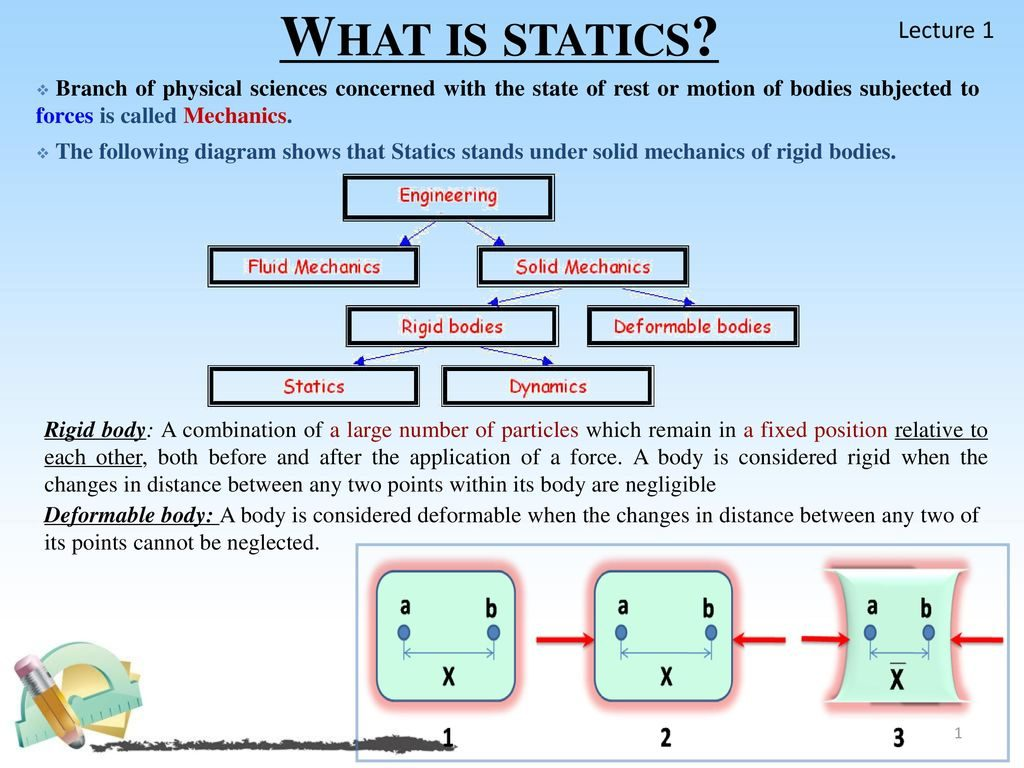Branch of physical sciences concerned with the state of rest or motion of bodies subjected to forces is called Mechanics. The following diagram shows that Statics stands under solid mechanics of rigid bodies. Rigid body: A combination of a large number of particles which remain in a fixed position relative to each other, both before and after the application of a force. A body is considered rigid when the changes in distance between any two points within its body are negligible. Deformable body: A body is considered deformable when the changes in distance between any two of its points cannot be neglected.

Statics assumes that the bodies with which it deals are perfectly rigid. It also holds that the sum of all the forces acting on a body at rest has to be zero (i.e., the forces involved balance one another) and that there must be no tendency for the forces to turn the body about any axis. These three conditions are independent of one another, and their expression in mathematical form comprises the equations of equilibrium. There are three equations, and so only three unknown forces can be calculated. If more than three unknown forces exist, it means that there are more components in the structure or machine than are required to support the applied loads or that there are more restraints than are needed to keep the body from moving. Such unnecessary components or restraints are termed redundant (e.g., a table with four legs has one redundant leg) and the system of forces is said to be statically indeterminate. The number of equations available in statics is limited because of a neglect of the deformations of loaded bodies, a direct consequence of the underlying premise that any solid body under consideration is ideally rigid and immutable as to shape and size under all conditions.

Fluid Flow

Properties of Fluids

Definition of a Fluid

Liquids and gases lack the ability of solids to offer a permanent resistance to a deforming force

– they deform continuously for as long as the force is applied. Deformation is caused by shearing forces which act tangentially to the surfaces to which they are applied.

A fluid is a substance which deforms continuously under the action of shearing forces, however small

Corollary:   for a fluid at rest ⇒ must no shearing forces

• all forces must be perpendicular to planes upon which they act

ViscocityDeformation (shear strain) of a notional element of fluid under the action of a shear stress (i.e. shear force per unit area).

For a solid, a given shear stress τ results in a given, fixed shear strain φ , i.e. φ τ

For a liquid, however, it turns out that it is the rate of change of strain that is proportional to the applied shear stress;

 ∂ϕ ∝ τ ∂t

From Figure 1.2, when φ is small, φ ≈ x / y

 ⇒ ∂ϕ = ∂  x = 1 ∂x = u ∂t y ∂ty ∂t y

where u is the velocity in the x direction.

 ⇒ τ ∝ ∂φ ∝ u ⇒ τ = const × u ∂t y y

 ⇒ τ = µ du NEWTON’S LAW OF VISCOSITY dy dynamic viscosity shear stress (Nm-2) (kgm-1s-1)

Thus, viscosity is a property of a fluid which tells us how easy (or difficult) it is to shear the fluid, i.e. how easily will it flow under given shearing forces.

Fluids obeying Newton’s Law of Viscosity are known as Newtonian Fluids.

Non-Newtonian fluids include “plastic fluids” such as sewage sludge and toothpaste and “visco-elastic fluids” such as custard…

Shearing forces within the body of a fluid arise due to adjacent “layers” of fluid moving past each other, e.g. near a wall;Viscosity is a measure of the fluid friction between adjacent (notional) layers of the flowing fluid.

Intuitively,                           µgases << µliquids

For gases, friction (viscosity) is due to inter-molecular interactions / collisions;

µgas  ↑  as temperature T

In liquids, friction (viscosity) is due to intermolecular cohesion, so

µliquid  ↓ as temperature T

E.g. the viscosity of water decreases by a factor of 3 between temperatures of 5 0C and 55 0C.

Note: the combination µ / ρ occurs sufficiently often in Fluid Mechanics equations that it is given its own symbol (ν, “nu”) and name (kinematic viscosity). FM2 will use dynamic viscosity, µ as this is the more fundamental and physically “tangible” measure, but you will see ν elsewhere, so you need to know about it!

Density

density = mass per unit volume, i.e. ρ = m / V

specific density  or specific gravity  ρ / ρwater   (with ρwater  taken as 1000 kgm-3).

Flows in which variations in ρ are negligible are termed incompressible

Flows in which variations in ρ are non-negligible are termed compressible

A working definition of negligible might be < 5%.

• for our purposes, all liquid flows may be regarded as incompressible
• gas flows in which the flow speed u « sound speed cs show only small variations in density and may also be regarded as incompressible.

u / cs M      the Mach number

for Mc. 0.3, Δρ / ρc. 5%

Surface Tension (liquids only)

Within the body of the liquid, inter-molecular attractive forces on average cancel out.

At an interface, upward and downward attractions may be unbalanced. The liquid surface behaves like an elastic membrane under tension.

• surface tension σ = force in liquid surface perpendicular to a line of unit length in the surface.
• σ is constant over the surface of separation (at a given temperature)
• as T ↑ , σ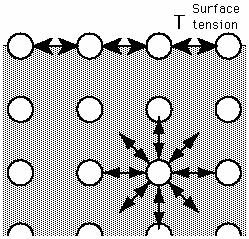The effect of surface tension is to reduce the free surface area of a body of liquid to a minimum, e.g. droplets.

Surface tension forces are often small in engineering flows, so surface tension is often neglected (or forgotten). The times when it is important to remember it are when dealing with fluids problems involving droplets and/or bubbles, where it can be very important in determining key aspects of the flow (e.g. droplet break-up in a spray).

Beware: Surface tension effects can cause problems when using scaled flow models.

Basic Flow Fields and Phenomena

Laminar and Turbulent Flows

Laminar flow

• characterised by smooth fluid motion in “layers” (“laminae”)
• dye injected appears as a single line
• velocity may vary from layer to layer

Turbulent flow

• characterized by random, 3-d motion (generally in addition to a mean flow)
• dye injected breaks up into myriad of entangled threads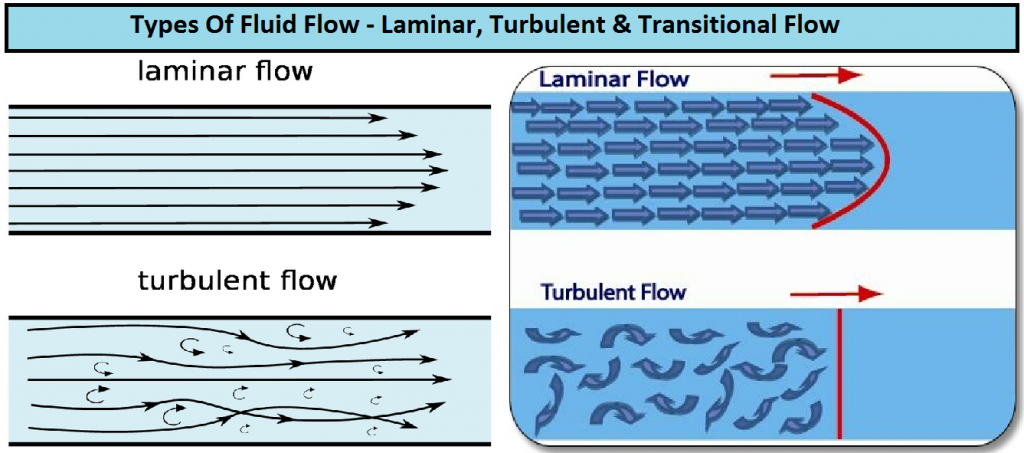Most (but certainly not all) flows of engineering interest are turbulent. Understanding turbulence is one of the most difficult areas of Fluid Mechanics. While many useful (simplifying) models have been developed over the last 60 years or so, there remain significant unsolved problems associated with turbulence.

Osborne Reynolds studied flows in a tube and found that the regime – laminar or turbulent – was determined by the parameter.

Re is the Reynolds number, D is the diameter of the pipe, and ρ, µ and u have their usual meanings. Note that ν is the kinematic viscosity ≡ µ

For flow in a pipe, we can identify three flow regimes:

Re < c. 2000                   laminar flow (if undisturbed)

Re > c. 4000                   turbulent flow

2000 < Re < 4000         transitional  – could be either laminar or turbulent

(can’t tell from Re alone)

Note that in transitional regime, at any point and time, the flow will be either laminar or turbulent. Physically, a flow cannot be both laminar and turbulent simultaneously, but it may switch between regimes, apparently randomly (e.g. due to a small disturbance to flow).

Re is a key parameter in many flow problems (probably the single most-quoted parameter in Fluid Mechanics).

At low Re, viscous forces dominate

At high Re, viscous forces become unimportant (and can sometimes be neglected)

Flow in an Enclosed Duct / Pipe

MECHANICAL PROPERTIES OF MATTER

Density

Density is a property of every substance which is mass unit per volume.Weight Density.

Weight Density is the weight of the substance per unit volume.Specific Gravity.

Specific Gravity of a substance is it’s density relative to that of water and is a pure number.

Specific Gravity is also called Relative DensityElasticity

Stress is the magnitude of force applied in a body divided by the area over it is exerted.Hooke’s Law

Hooke’s Law states that the amount of deformation experienced by a body under stress is proportional to the magnitude of the force applied to it.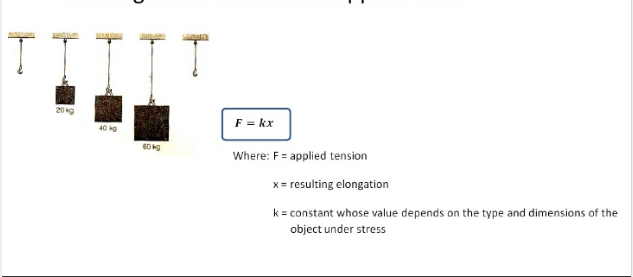Properties Of Materials

Ductility is the property of metals which can be deformed beyond the elastic limit.

Malleability is the property of metals which has a large plastic range in compression.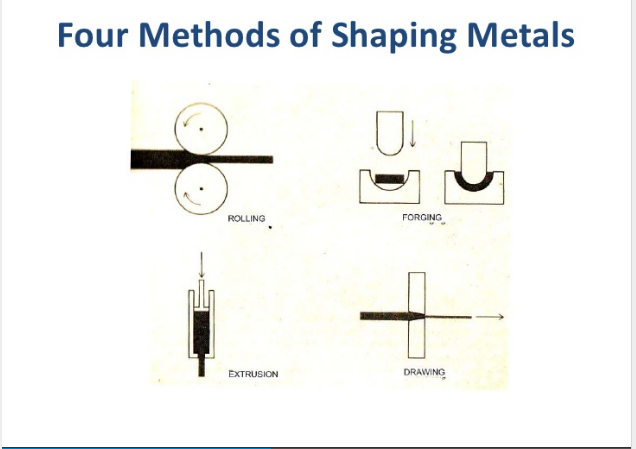Structure Of Solids.

Crystalline is the structure of solids in which atoms are arranged in regular pattern.Stress is defined as the ratio of applied force over area.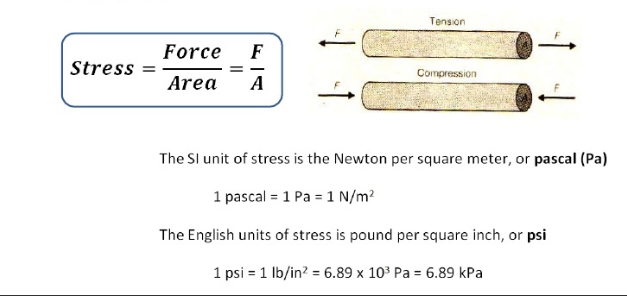Strain is equal to the ratio between the change in length and the original length.Modulus Of Elasticity is defined as the ratio between the stress and the strain.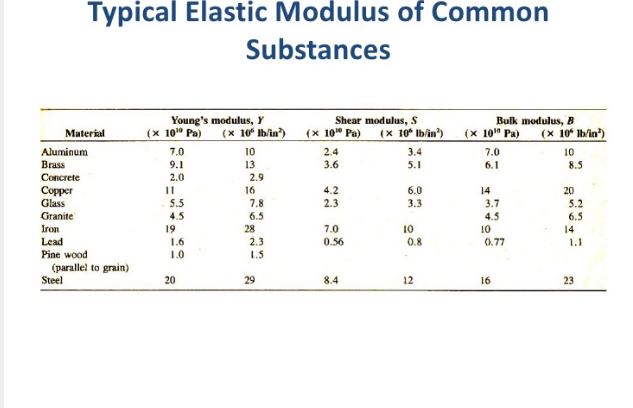Shear

Shear stresses change the shape of an object on which they act but not on it’s volume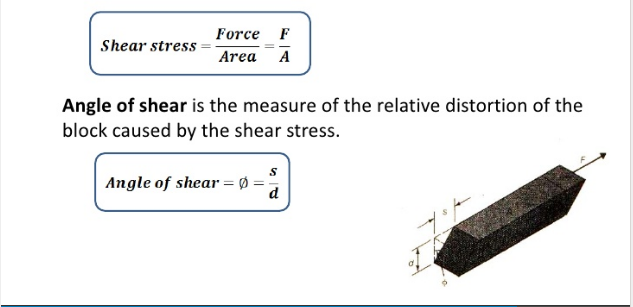Uniform Motion In A Circle.

DEFINITION OF UNIFORM CIRCULAR MOTION

Uniform circular motion is the motion of an object traveling at a constant speed on a circular path.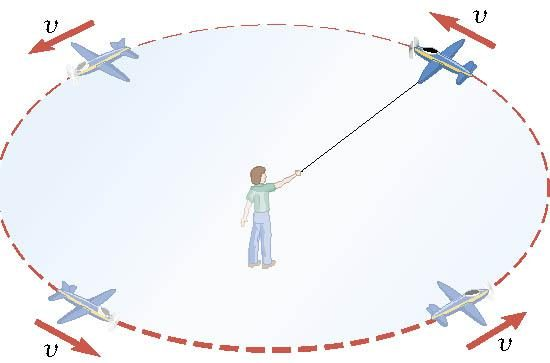Example 1: A Tire-Balancing Machine

The wheel of a car has a radius of 0.29m and it being rotated at 830 revolutions per minute on a tire-balancing machine. Determine the speed at which the outer edge of the wheel is moving.

 1 = 1.2 “10!3 min revolution 830 revolutions min

• =2 !103 min = 0.072 s

• = 2! r = 2! (29 m) = 25 m s

T0.072 s

In uniform circular motion, the speed is constant, but the direction of the velocity vector is not constant.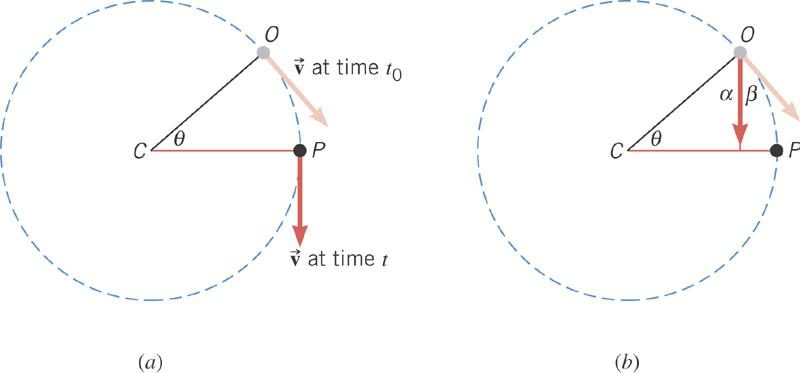The direction of the centripetal acceleration is towards the center of the circle; in the same direction as the change in velocity.

Conceptual Example 2: Which Way Will the Object Go?

An object is in uniform circular motion. At point O it is released from its circular path. Does the object move along the straight path between O and A or along the circular arc between points O and P ?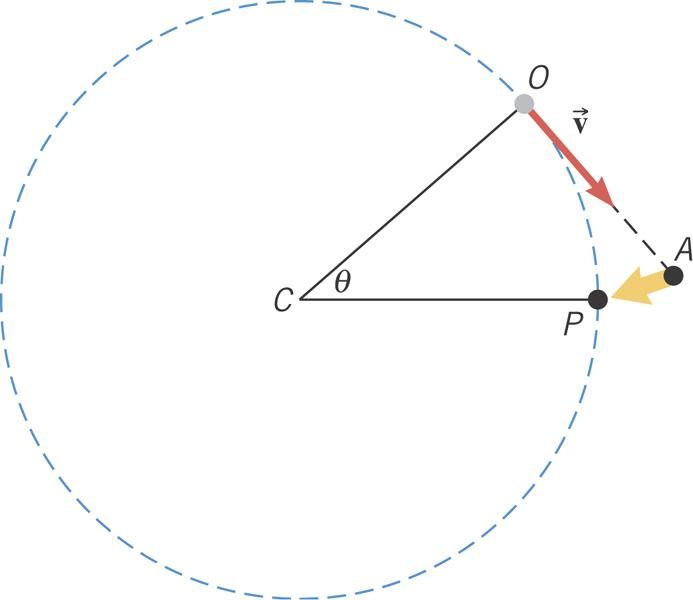Centripetal Force

When a net external force acts on an object of mass m, the acceleration that results is directly proportional to the net force and has a magnitude that is inversely proportional to the mass. The direction of the acceleration is the same as the direction of the net force.

Thus, in uniform circular motion there must be a net force to produce the centripetal acceleration.

The centripetal force is the name given to the net force required to keep an object moving on a circular path.

The direction of the centripetal force always points toward the center of the circle and continually changes direction as the object moves.

v2

Fc = mac = m r

The Effect of Speed on Centripetal Force

The model airplane has a mass of 0.90 kg and moves at constant speed on a circle that is parallel to the ground. The path of the airplane and the guideline lie in the same horizontal plane because the weight of the plane is balanced by the lift generated by its wings. Find the tension in the 17 m guideline for a speed of 19 m/s.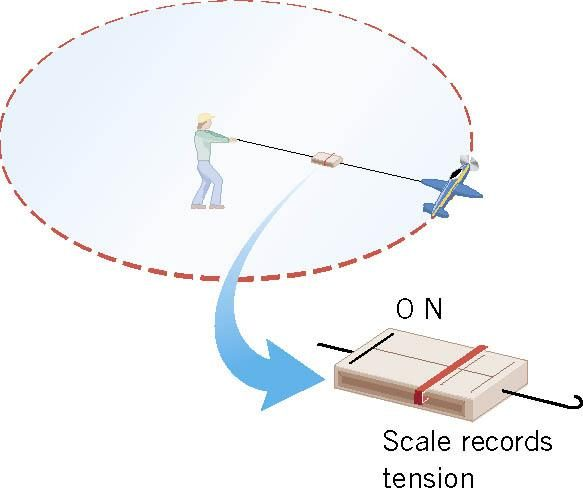v2

Fc = T = m r

• = (90 kg)(19 ms)2 = 19 N 17 m

Conceptual Example 6: A Trapeze Act

In a circus, a man hangs upside down from a trapeze, legs bent over and arms downward, holding his partner. Is it harder for the man to hold his partner when the partner hangs straight down and is stationary of when the partner is swinging through the straight-down position?Vertical Circular Motion

In vertical circular motion the gravitational force must also be considered.

An example of vertical circular motion is the vertical “loop-the-loop” motorcycle stunt. Normally, the motorcycle speed will vary around the loop.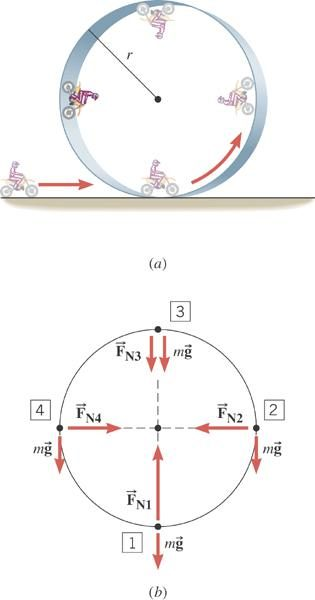The normal force, FN, and the weight of the cycle and rider, mg, are shown at four locations around the loop.

Gravitation

Newton’s Law of Gravitation

Every particle of matter in the universe attracts every other particle with a force that is directly proportional to the product of the masses of the particles and inversely proportional to the square of the distance between them.

Mathematically, this law, and the magnitude of the force due to the gravitational interaction between two particles, is expressed with

 F = Gm1m2 , (5.1) r2 grav where  G = 6.67 × 10−11 N ⋅ m2 /kg2   is the universal gravitational constant, m1  and m2

the particles masses, and r the distance between them. Equation (5.1) is however not complete since the force due to gravitation is vectorial in nature. If we define the vectors r1 and r2 for the positions of m1 and m2 , respectively, and the unit vector that points

from r1  to r2  is er  = (r2r1 ) r2 r1 , then the force that m2  exerts on m1 is

F21 = Fgraver

 = Gm1m2 e . (5.2) r2 r Conversely, accordingly to Newton’s Third Law, the force that m1  exerts on m2  is F12  = −F21 Gm1m2 e (5.3) = − . r2 r

Gravitational Interactions with Spherically Symmetric Bodies

Although the related calculations are beyond the scope of our studies, it can be shown that gravitational interactions involving spherically symmetric bodies (e.g., uniform spheres, cavities, and shells) can be treated as if all the mass was concentrated at the centres of mass of the bodies . This property is especially useful when dealing with the earth, other planets and satellites, as well as the sun and stars, which can all be approximated as being spherically symmetric.

There are other interesting results that can be derived for spherically symmetric bodies. For example, the gravitational force felt by a mass m located at a radius r inside a spherical shell of radius R and mass M (i.e., with rR ) is zero. That is,

 0, r < R Fgrav GmM (5.4) = er , − r ≥ R r 2

with  er            the unit vector directed from the centre of the shell to the mass. It therefore follows from this that the gravitational force felt by a mass located inside/outside a uniform spherical shell can be shown to be equal to the portion of the mass of the sphere contained within the radius r . Mathematically this can expressed withThe result when the mass is located inside the sphere, i.e., when r < R , can be explained by considering the relation between the mass M , the mass density ρ (units of kg/m3 ), and the volume V (units of m3 ) of the sphere. More precisely, we have that

 M = ρV,

with

 V = 4 π R3 (5.7) 3

for a sphere. If the sphere is uniform, then the density ρ is constant throughout. It follows that the mass Mr contained within a volume Vr (with a radius rR ) is

 Mr = ρVr 4 π r 3 = ρ 3 r  3 4 3 = ρ π R 3 R r  3 = ρV , R

or from equation (5.6)

 r 3 Mr = M . R

We can then use equation (5.3) to write down the force felt by the mass when it is located inside the sphere (when rR )

 F = − GmMr e grav r2 r 3 (5.10) GmM r = − er r 2 R

and

 F = − GmMr e . (5.11) grav R3r

Weight and Gravitational Potential Energy

In previous chapters we modeled the force exerted by the earth on a particle of mass m by its weight

 w = mg,

with g the gravitational acceleration due to the earth. Referring to Problem 2 above, we can now easily evaluate this quantity by equating the weight with the gravitational force exerted by the earth at its surface. That is,

 mg = GmE m , (5.18) R2 E

or

g = GmE

RE2

= 6.67 × 1011 Nm2 /kg25.97 × 1024 kg

(5.19)

(6.37 × 106 m)2

• 81 m/s2 ,

as was expected. It should however be clear, and it is important to note, that the gravitational acceleration and force exerted by the earth vary with the position of the particle above the surface of the earth; they both decrease as the particle is located further away from the surface (or the centre) of the earth.

We also saw that the gravitational force is conservative. That is, the change of potential energy associated with the gravitational force is only a function of the initial and final positions of the path traced by the particle. This in turn means that the gravitational force is (minus) the gradient of the gravitational potential energy Ugrav

 Fgrav = −∇Ugrav .

For a particle located at a position rRE  the gravitational force exerted by the earth is

 F = − GmE m e , grav r2r

where m is once again the mass of the particle and er is the unit vector pointing from the center of the earth to the position occupied by the particle. Combining equations  and  we write

 dUgrav = GmE m . (5.22) dr r2

It can readily be verified that

 Ugrav = − GmE m r

is a solution consistent with equations (5.20) and (5.22). Equation (5.23) is however different to the relation we have used so far for the gravitational potential energy

 Ugrav = mg r,

where the quantity r can be conveniently chosen to be the position of the particle relative to the surface of the earth. We will now establish that this apparent disparity is solely due to our previously implied assumption that the particle is located very near the surface of the earth, where the gravitational acceleration can, to a good approximation, considered constant at g .

Let us then assume that

 r = RE +  r,

with     r        RE .

 Ugrav = − GmE m RE + r = − GmE m RE (1 + r RE ) − GmE m 1 − r , RE RE

since when     r        RE

 1 1 − r . ( 1 +  r RE RE

We now modify equation  to

 GmE m GmE r. Ugrav − + m RE 2 RE

We finally make two observations: i) the quantity in parentheses in the second term on the right-hand side of equation (5.28) is simply the gravitational acceleration at the surface of the earth (see equation (5.19)) and ii) as was stated previously in Section 2.7 of Chapter 2 the potential gravitational energy can only been defined up to constant, and thus the first term on the right-hand side of equation (5.28), which is constant, can be ignored (if one wishes) and the potential gravitational energy near the surface of the earth redefined to

 Ugrav     mg r.

This justifies the relation we have used so far.

Referring back to our analysis  we could have easily derived equation for the gravitational potential energy by considering the work done by the gravitational force on the particle (of mass m ). That is, when the particle moves from a point r1 to another point at r2 in the earth’s gravitational field we have

 W = ∫12 Fgrav ⋅ dr = −GmE m∫12 er ⋅ dr r2 2 dr (5.30) = −GmE m∫1 r2 = GmE m − GmE m , r2 r1

since ∫ drr2 = −1 r .

 W = Ugrav (r1 ) −Ugrav (r2 ),

which is perfectly consistent with the corresponding equation we derived in S. This once again verifies that the gravitational force is indeed conservative, since the change in potential energy is not a function of the path taken between the initial and final points. Although these results were derived using the gravitational interaction with the earth as an example, they apply to any body in general.

Kepler’s Laws

Several decades before Newton enunciated his Law of Gravitation, Johannes Kepler used precise astronomical data of planetary motions to empirically deduce three general laws governing the orbits of the planets orbiting the sun. These three laws are as follows:

1. Planets move on elliptical orbits about the sun with the sun at one focus.

1. The area per unit time swept out by a radius vector from the Sun to a planet is constant.
• The square of a planet’s orbital period is proportional to the cube of the major axis of the planet’s orbit.

These laws were all, in time, rigorously explained by Newton. But the derivation of the proof of Kepler’s First Law, in particular, requires a level of mathematical sophistication that is beyond the scope of our studies. An example of an elliptical planetary orbit is shown in Figure 5, where the sun is located at the focus S . The orbit is characterized by its semi-major axis a , which is a measure of the size of the orbit, and its eccentricity e . The distance of the two foci from the centre of the ellipse is given by ±ea on the major axis line. It is interesting to note that the orbits of planets in the solar system have relatively modest eccentricities, ranging from 0.007 for Venus and 0.206 for Mercury. The earth has an eccentricity of 0.017, while an eccentricity of zero corresponds to a circular orbit. The closest and furthest orbital points to the sun cross the major axis at the perihelion and the aphelion, respectively.

For Kepler’s Second Law we can start by considering the torque acting on the planet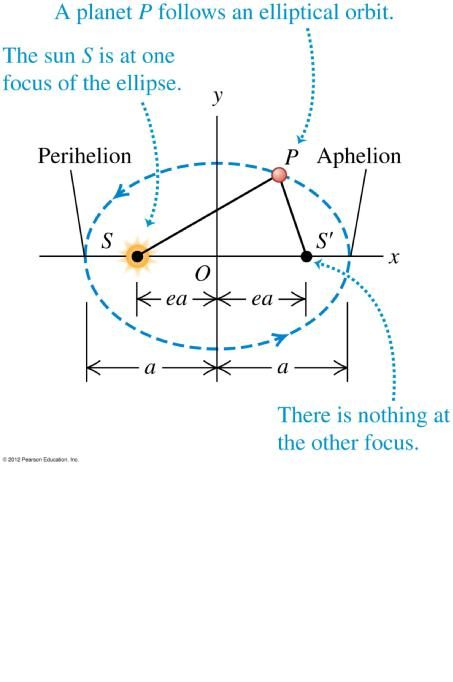τ = r × Fgrav , (5.41)

where also, as usual, τ = dL dt .

However, since the vector r defining the position of the planet in relation to the sun and the gravitational pull of the sun on the planet Fgrav are collinear, the torque on the planet is zero. It follows that the angular momentum of the planet is conserved.

Considering the definition for the angular momentum

L = r × p

 = mrvsin(φ)e⊥ = constant,

where e is the unit vector perpendicular to orbital plane, m is the planet’s mass, and φ is the angle between planet’s linear momentum vector p and r (see Figure 6). Since the orbital motion takes place in a plane, we can use

 v⊥ ≡ v sin (φ) = ωr, to transform equation (5.42) to L = r2ω m = r2 dθ , dt since  ω = dθ dt .  However,  the  triangular  area  dA  swept  as  the  planet travels  an infinitesimal angle dθ is one-half the product of the radius r and the arc rdθ dA = 1 r2dθ.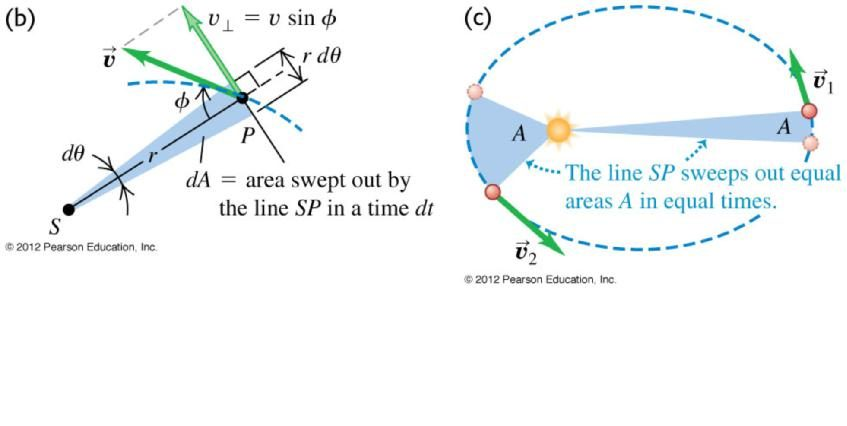## STEM Elearning

We at FAWE have built this platform to aid learners, trainers and mentors get practical help with content, an interactive platform and tools to power their teaching and learning of STEM subjects, more

#### How to find your voice as a woman in Africa

© FAWE, Powered by: Yaaka DN.